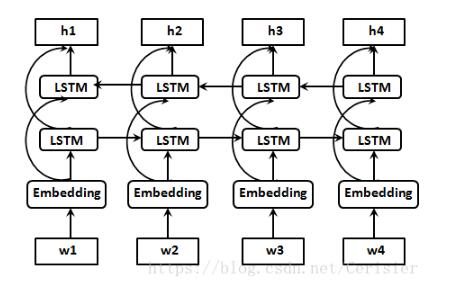# 双向RNN:bidirectional_dynamic_rnn()函数的使用详解``````
tf.nn.bidirectional_dynamic_rnn(
cell_fw,
cell_bw,
inputs,
sequence_length=None,
initial_state_fw=None,
initial_state_bw=None,
dtype=None,
parallel_iterations=None,
swap_memory=False,
time_major=False,
scope=None
)``````

``````
with vs.variable_scope(scope or "bidirectional_rnn"):
# Forward direction
with vs.variable_scope("fw") as fw_scope:
output_fw, output_state_fw = dynamic_rnn(
cell=cell_fw, inputs=inputs,
sequence_length=sequence_length,
initial_state=initial_state_fw,
dtype=dtype,
parallel_iterations=parallel_iterations,
swap_memory=swap_memory,
scope=fw_scope)``````

``````
with vs.variable_scope("bw") as bw_scope:
# ———————————— 此处是重点 ————————————
inputs_reverse = _reverse(
inputs, seq_lengths=sequence_length,
seq_dim=time_dim, batch_dim=batch_dim)
# ————————————————————————————————————
tmp, output_state_bw = dynamic_rnn(
cell=cell_bw,
inputs=inputs_reverse,
sequence_length=sequence_length,
initial_state=initial_state_bw,
dtype=dtype,
parallel_iterations=parallel_iterations,
swap_memory=swap_memory,
time_major=time_major,
scope=bw_scope)``````

``````
outputs = (output_fw, output_bw)
output_states = (output_state_fw, output_state_bw)``````